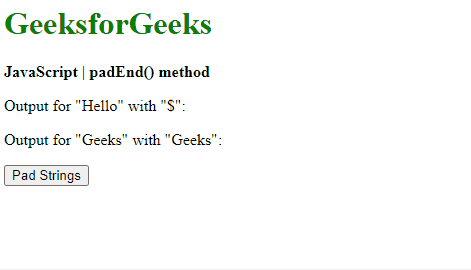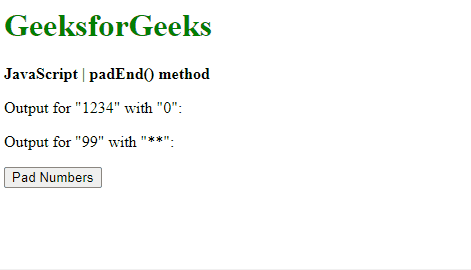Open in App
Not now

• Last Updated : 26 Dec, 2022

The padEnd() method in JavaScript is used to pad a string with another string until it reaches the given length. The padding is applied from the right end of the string.

Syntax:

`string.padEnd( targetLength, padString )`

Parameters: This method accepts two parameters as mentioned above and described below:

• targetLength: It is the length of the final string once the original string has been padded. If the value is less than the original string length, then the original string is returned.
• padString: It is the string that is to be padded with the original string. If this value is too long to be within the targetLength, it is truncated.

Return Value: It returns the final string that is padded with the given string of the given length.

Example 1: This example uses the padEnd() method to pad strings into another string.

## html

 `<``h1` `style``=``"color: green"``>``    ``GeeksforGeeks```` ` `<``b``>``    ``JavaScript | padEnd() method```` ` `<``p``>``    ``Output for "Hello" with "\$":``    ``<``span` `class``=``"output"``>```` ` `<``p``>``    ``Output for "Geeks" with "Geeks":``    ``<``span` `class``=``"output2"``>```` ` `<``button` `onclick``=``"padStrings()"``>``    ``Pad Strings```` ` `<``script` `type``=``"text/javascript"``>``    ``function padStrings() {``        ``exString = "Hello";``        ``exString2 = "Geeks";``     ` `        ``output =``            ``exString.padEnd(12, "\$");``        ``output2 =``            ``exString2.padEnd(12, "Geeks");``     ` `        ``document.querySelector('.output').textContent``                ``= output;``         ` `        ``document.querySelector('.output2').textContent``                ``= output2;``    ``}```

Output:Example 2: This example uses the padEnd() method to pad numbers into another number.

## html

 `<``h1` `style``=``"color: green"``>``    ``GeeksforGeeks```` ` `<``b``>``    ``JavaScript | padEnd() method```` ` `<``p``>``    ``Output for "1234" with "0":``    ``<``span` `class``=``"output"``>```` ` `<``p``>``    ``Output for "99" with "**":``    ``<``span` `class``=``"output2"``>```` ` `<``button` `onclick``=``"padNumbers()"``>``    ``Pad Numbers```` ` `<``script` `type``=``"text/javascript"``>``    ``function padNumbers() {``        ``exNumber = 1234;``        ``exNumber2 = 99;``     ` `        ``output =``            ``String(exNumber).padEnd(8, "0");``             ` `        ``output2 =``            ``String(exNumber2).padEnd(8, "**");``             ` `     ` `        ``document.querySelector('.output').textContent``                ``= output;``         ` `        ``document.querySelector('.output2').textContent``                ``= output2;``    ``}```

Output:We have a complete list of Javascript Strings, to check those please go through the Javascript String Complete reference article.

Supported Browsers: The browser supported by the padEnd() method are listed below: